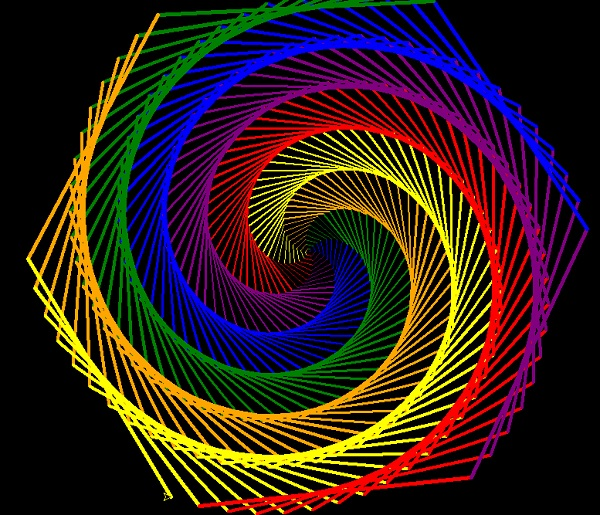# Turtle programming in Python

Turtle is a special feature of Python. Using Turtle, we can easily draw in a drawing board.

First, we import the turtle module. Then create a window, we create a turtle object, and using the turtle() method we can draw on the drawing board.

## Some turtle method

METHOD PARAMETER DESCRIPTION
Turtle() None It creates and returns a new turtle object
forward() amount It moves the turtle forward by the specified amount
backward() amount It moves the turtle backward by the specified amount
right() angle It turns the turtle clockwise
left() angle It turns the turtle counter clockwise
penup() None It picks up the turtle’s Pen
pendown() None Puts down the turtle’s Pen
up() None Picks up the turtle’s Pen
down() None Puts down the turtle’s Pen
color() Color name Changes the color of the turtle’s pen
fillcolor() Color name Changes the color of the turtle will use to fill a polygon
position() None It returns the current position
goto() x, y It moves the turtle to position x,y
begin_fill() None Remember the starting point for a filled polygon
end_fill() None It closes the polygon and fills with the current fill color
dot() None Leaves the dot at the current position
stamp() None Leaves an impression of a turtle shape at the current location
shape() shapename Should be ‘arrow’, ‘classic’, ‘turtle’ or ‘circle’

## Example code

# import turtle library
import turtle
my_window = turtle.Screen()
my_window.bgcolor("blue")       # creates a graphics window
my_pen = turtle.Turtle()
my_pen.forward(150)
my_pen.left(90)
my_pen.forward(75)
my_pen.color("white")
my_pen.pensize(12)

## Output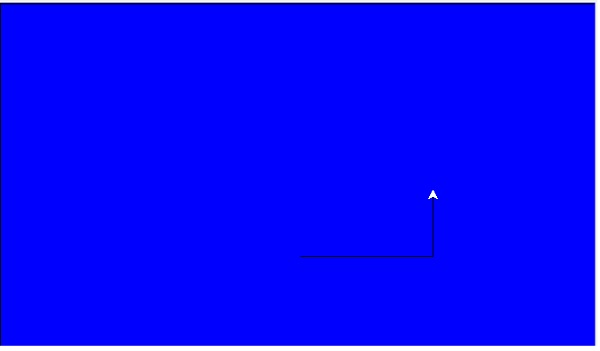## Example code

# import turtle library
import turtle
my_pen = turtle.Turtle()
for i in range(4):
my_pen.forward(50)
my_pen.right(90)
turtle.done()

## Output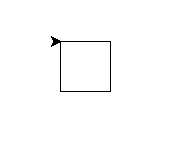## Example code

# import turtle library
import turtle
my_pen = turtle.Turtle()
for i in range(50):
my_pen.forward(50)
my_pen.right(144)
turtle.done()

## Output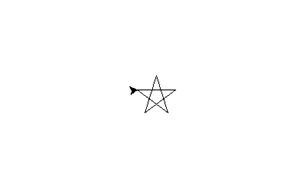## Example code

# import turtle library
import turtle
polygon = turtle.Turtle()
my_num_sides = 6
my_side_length = 70
my_angle = 360.0 / my_num_sides
for i in range(my_num_sides):
polygon.forward(my_side_length)
polygon.right(my_angle)
turtle.done()

## Output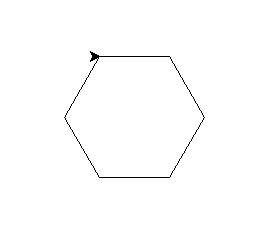## Example code

# import turtle library
import turtle
my_wn = turtle.Screen()
my_wn.bgcolor("light blue")
my_wn.title("Turtle")
my_pen = turtle.Turtle()
my_pen.color("black")
def my_sqrfunc(size):
for i in range(4):
my_pen.fd(size)
my_pen.left(90)
size = size - 5
my_sqrfunc(146)
my_sqrfunc(126)
my_sqrfunc(106)
my_sqrfunc(86)
my_sqrfunc(66)
my_sqrfunc(46)
my_sqrfunc(26)

## Output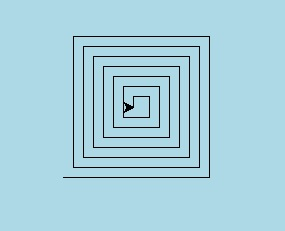## Example code

# import turtle library
import turtle
my_wn = turtle.Screen()
turtle.speed(2)
for i in range(30):
turtle.circle(5*i)
turtle.circle(-5*i)
turtle.left(i)
turtle.exitonclick()

## Output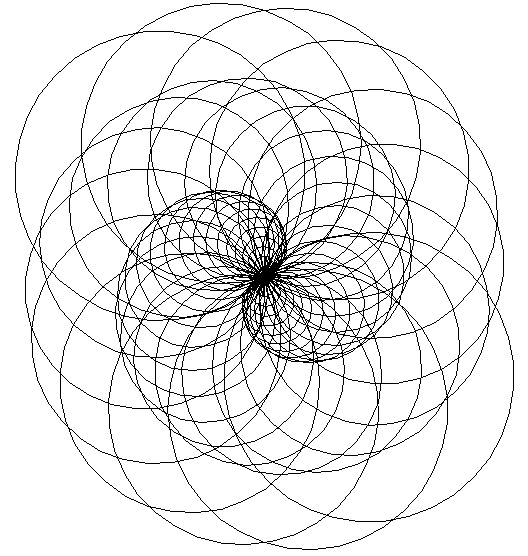## Example code

# import turtle library
import turtle
colors = [ "red","purple","blue","green","orange","yellow"]
my_pen = turtle.Pen()
turtle.bgcolor("black")
for x in range(360):
my_pen.pencolor(colors[x % 6])
my_pen.width(x/100 + 1)
my_pen.forward(x)
my_pen.left(59)

## Output